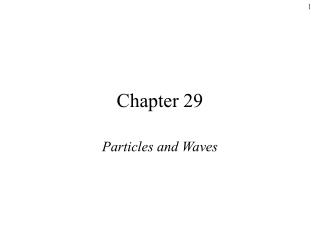Download PresentationChapter 29

# Chapter 29

Download Presentation## Chapter 29

- - - - - - - - - - - - - - - - - - - - - - - - - - - E N D - - - - - - - - - - - - - - - - - - - - - - - - - - -
##### Presentation Transcript

1. Chapter 29 Particles and Waves

2. There is nothing new to be discovered in physics now. All that remains is more and more precise measurement. -- William Thomson, Lord Kelvin(Address at the British Association for the Advancement of Science, 1900).

3. Quantum Physics • 2nd revolution in physics: • Starts with Planck ~1900 • Contributions from Einstein, Bohr, Heisenberg, Schrödinger, Born, Dirac, de Broglie …. over 25 years • Cornerstones: • Wave-particle duality • Uncertainty principle • Correspondence • Applies for small dimensions • Planck’s constant: h = 6.6 x 10-34Js • As h -> 0, quantum physics -> classical

4. Solvay conference, 1927 Front row: I. Langmuir, M. Planck, M. Curie, H. A. Lorentz, A. Einstein, P. Langevin, C. E. Guye, C. T. R. Wilson, O. W. Richardson. Second row: P. Debye, M. Knudsen, W. L. Bragg, H. A. Kramers, P. A. M. Dirac, A. H. Compton, L. V. de Broglie, M. Born, N. Bohr. Standing: A. Piccard, E. Henriot, P. Ehrenfest, E. Herzen, T. De Donder, E. Schroedinger, E. Verschaffelt, W. Pauli, W. Heisenberg, R. H. Fowler, L. Brillouin.

5. Solvay conference, 1927 Heisenberg Schrödinger deBroglie Compton Pauli Born Bohr Dirac Bragg Lorentz Planck Curie Einstein Front row: I. Langmuir, M. Planck, M. Curie, H. A. Lorentz, A. Einstein, P. Langevin, C. E. Guye, C. T. R. Wilson, O. W. Richardson. Second row: P. Debye, M. Knudsen, W. L. Bragg, H. A. Kramers, P. A. M. Dirac, A. H. Compton, L. V. de Broglie, M. Born, N. Bohr. Standing: A. Piccard, E. Henriot, P. Ehrenfest, E. Herzen, T. De Donder, E. Schroedinger, E. Verschaffelt, W. Pauli, W. Heisenberg, R. H. Fowler, L. Brillouin.

6. Part I: Particle nature of light

7. Cavity is model of a perfect blackbody 1. Blackbody radiation a) Blackbody • All objects radiate and absorb electromagnetic radiation • At equilibrium, rate of absorption = rate of emission • Best absorber is best emitter • Perfect absorber is perfect emitter

8. b) Emmitance spectrum: the problem Plot of intensity vs wavelength • Depends only on temperature Experimental spectrum:

9. Theory Classical prediction: The UV catastrophe • Based on idea that all oscillations equally probable, more oscillations at lower wavelength • Violates common sense and experiment

10. c) Energy quantization: the solution Absorption and emission occur in discrete quanta only Energy of quanta proportional to frequency • For small wavelength (high freq), quanta are large. If kT < quantum, radiation not possible.

11. d) Planck’s constant, h • Planck found a “fudge factor” by “happy guesswork” to make the experiment fit. He developed a quantization theory to predict the value h. • “lucky artifact of more fundamental reality yet to be discovered” • Nobel prize, 1918

12. 2. Photoelectric effect a) The effect

13. b) Expectations and observations

14. Observed frequency dependence

15. b) Expectations and observations

16. Work required to remove electron Energy of photon (from Planck) c) Einstein theory KEmax = hf - W0

17. • Found same value for h as Planck had • Nobel prize in 1921 • In 1913, Planck recommended Einstein for membership in the Prussian Academy. “Notwithstanding his genius, he may sometimes have missed the target in his speculations, as, for example, in his hypothesis of light quanta.”

18. 3. The Compton Effect, 1923 The effect: Scattering of x-ray by electron changes the wavelength

19. X-ray source (l) Crystal q graphite Detector Bragg reflection gives l’ b) The experiment

20. c) Classical prediction - incident wave excites electron at frequency f - electron radiates at frequency f

21. l l’ d) Compton’s explanation - Conservation of energy: - Conservation of Momentum: - Energy-momentum relation for light: Combining these equations gives: Nobel prize, 1928 Definitive evidence for photons

22. Part II: The wave nature of particles

23. 4. The de Broglie wavelength, 1924 The hypothesis The dual nature observed in light is present in matter: } A photon has energy From electromagnetism, By analogy, de Broglie proposed that a particle with momentum p is associated with a wave with wavelength:

24. b) Electron diffraction/interference

25. b) Interpretation of the particle wave Waves and particles propogate and interfere like waves, but interact like particles. The intensity of the wave (represented by a wave function at a point in space represents the probability of observing a particle at that location.

26. 5. The Heisenberg Uncertainty Principle The wave nature of particles means that position and momentum (wavelength) cannot simultaneously be determined to arbitrary accuracy. The smaller the slit above, the better the y-position is known, but the greater the spread in y-momentum.

27. The principle applies separately to any component of momentum and position: and to energy and time: